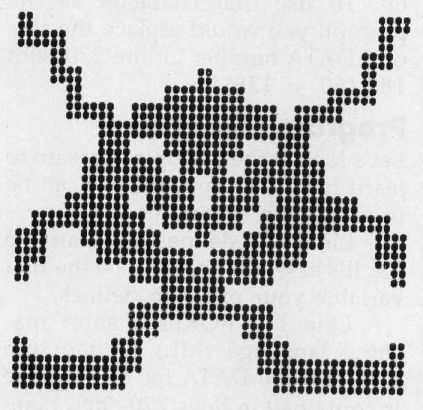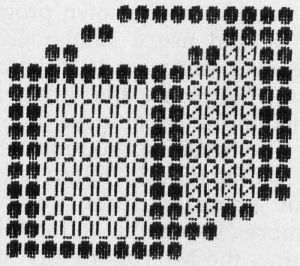`COMPUTE! ISSUE 61 / JUNE 1985 / PAGE 92`

 Hardcopy Sprites For Commodore 64 Thomas H. White Make a hardcopy printout of any Commodore 64 sprite with this BASIC program for the VIC-1525, MPS-801, or MPS-802 printer. It's easily adapted to other printers as well. Have you ever wished you could print a sprite on paper? For example, you may want to check some detail on a sprite you designed without bothering to load and run the program. With "Hardcopy Sprites," you can check such details in seconds rather than minutes, and even compile a personal library of sprite shapes for future reference. Ordinary screen dump programs can't make sprite printouts because the data that defines sprite shapes isn't located in regular screen memory. To print out a sprite, you need a program that reads the 64-byte memory area where the sprite shape is actually stored. Hardcopy Sprites uses this technique to print a 24 × 21 character representation of any sprite, with vertical or horizontal expansion if you wish. Large ball-shaped characters form single-color sprites, and additional characters represent multicolor sprites. Figures 1 and 2 show two examples of what is possible. After typing and saving the program, POKE your sprite data into memory and note the address where it begins. Then simply run Hard-copy Sprites and follow the prompts. The program asks you to enter the memory address where the sprite data begins, to give the sprite a descriptive name, to choose horizontal or vertical expansion (or both), and to select single-color or multicolor mode. Non-Commodore Printers Hardcopy Sprites is written for Commodore printers, but is readily adapted to other printers. If your printer can't handle Commodore graphics, replace the ball-shaped Commodore graphics character (SHIFT-Q in the program listing) in lines 200, 210, 230, 340, and 360 with some other character, such as an X or a plus sign. With a little extra work, you could also incorporate Hardcopy Sprites as a subroutine within a BASIC sprite editor program.Figure 1: Expanded Single-Color SpriteFigure 2: Unexpended Multicolor Sprite Hardcopy Sprites Please refer to "COMPUTE!'s Guide to Typing In Programs" before entering this listing. ```10 REM ** SPRITE HARDCOPY ** :rem 30 20 INPUT"{CLR}{DOWN}SPRITE DAT A MEMORY LOCATION";PG :rem 207 30 INPUT"{CLR}{DOWN}EXPAND X D IR. (Y/N)";EX\$ :rem 35 40 INPUT"{7 SPACES}Y DIR. (Y/N )";EY\$ :rem 194 45 INPUT"{DOWN}SPRITE NAME";N\$ :rem 243 47 INPUT"{DOWN}MULTICOLOR MODE (Y/N)";MS :rem 82 50 OPEN1,4 :rem 40 55 PRINT#1,CHR\$(15)"MEMORY LOC ATION"PG"{2 SPACES}"N\$ :rem 242 60 FORR=0TO60STEP3 :rem 132 70 FORC=0TO2:P=PEEK(PG+R+C) :rem 146 80 FORB=7TO0STEP-1:V(B)=P/2:P= INT(V(B)) :rem 111 90 V(B)=V(B)-INT(V(B)) :rem 202 100 IFV(B)>0THENGOSUB200 :rem 184 110 IFV(B)=0THENGOSUB250 :rem 189 120 NEXTB :rem 20 130 FORB=0TO7:L\$=L\$+D\$(B) :rem 129 140 NEXTB:NEXTC :rem 210 150 IFEY\$<>"Y"THENPRINT#1,CHR\$ (15)+CHR\$(16)+"28"+L\$+CHR\$ 8) :rem 255 160 IFEY\$="Y"THENFORRE=0TO1:PR INT#1,CHR\$(15)+CHRS(16)+"1 6"+L\$+CHR\$(8):NEXTRE :rem 201 170 L\$="":NEXTR :rem 84 180 PRINT#1:CLOSE1:END :rem 108 200 D\$(B)="Q" :rem 223 210 IFM\$="N"ANDEX\$="Y"THEND\$(B )="QQ":rem 29 220 IFM\$="Y"ANDEX\$="N"THENGOSU B300 :rem 19 230 IFM\$="Y"ANDEX\$="Y"THENDS(B )="QQ":GOSUB320 :rem 121 240 RETURN :rem 118 250 D\$(B)=" " :rem 19 260 IFM\$="N"ANDEX\$="Y"THEND\$(B )="{2 SPACES}":rem 128 270 IFM\$="Y"ANDEX\$="N"THENGOSU B340 :rem 28 280 IFM\$="Y"ANDEX\$="Y"THEND\$(B )="{2 SPACES}":GOSUB360 :rem 224 290 RETURN :rem 123 300 IFB=6ORB=4ORB=2ORB=0THENIF D\$(B+1)=" "THEND\$(B+1)="0" :D\$(B)="0":rem 128 310 RETURN :rem 116 320 IFB=6ORB=4ORB=2ORB=0THENIF D\$(B+1)="{2 SPACES}"THEND\$(B+1) = "00" :D\$(B) = "00" :rem 226 330 RETURN :rem 118 340 IFB=6ORB=4ORB=2ORB=0THENIF D\$(B+1)="Q"THEND\$(B+1)="O" :D\$(B) = "O" :rem 147 350 RETURN :rem 120 360 IFB=6ORB=4ORB=2ORB=0THENIF D\$(B+1)="QQ"THEND\$(B+1)="O O":D\$(B)="OO" :rem 4 370 RETURN :rem 122 ```